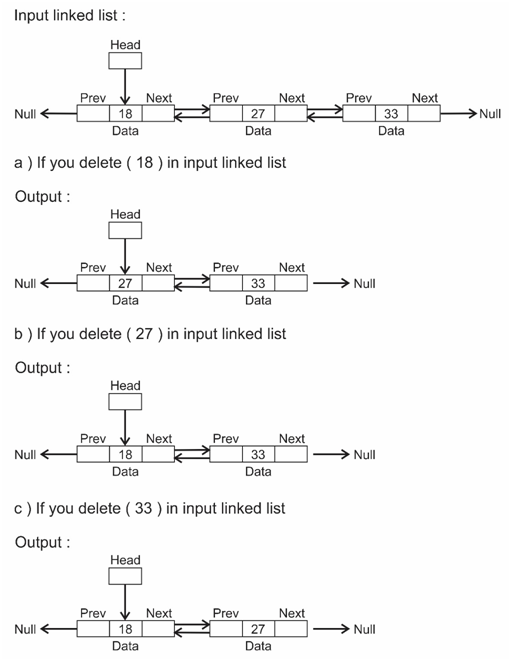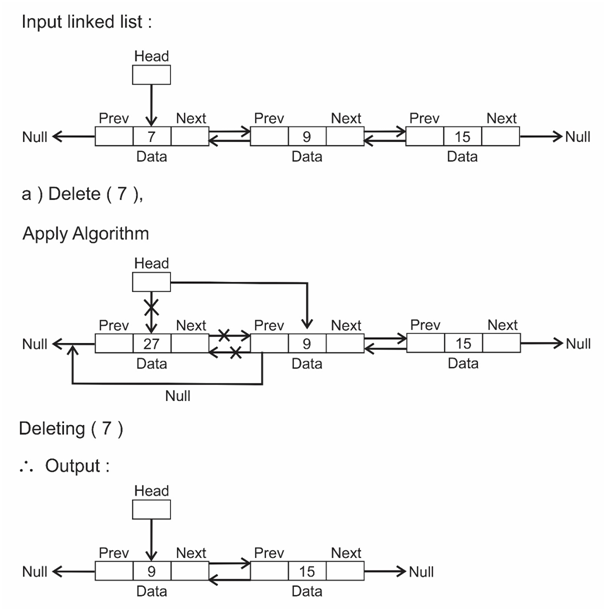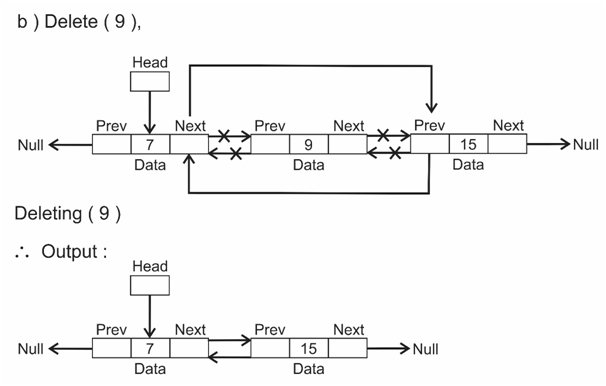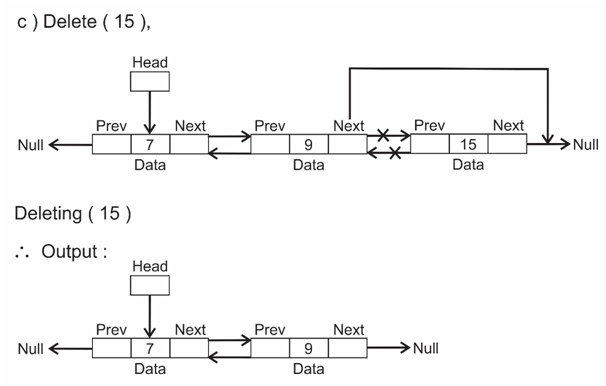# Delete a node in doubly linked list

## In the given doubly linked list, delete a node

We can delete head node, middle node or last node.

### Example## Algorithm

Time complexity : O(1)

Step 1 : create a function which takes a linked list and node that had to be deleted as arguments and delete the node.
Step 2 : If you want to delete a head node.
a)    Change the head pointer to next of current node (head here).
b)    Change the previous pointer of next node to current node previous.
Step 3 : If you want to delete middle node.
a)    Change the previous pointer of next node to current node previous
b)    Change the previous node next pointer to next of current node.
c)    Delete the node. (current node)
d)    If previous or next is NULL you need not delete them. (for deleting last node)
Step 4 : On the given linked list, call the function and give which node you want to delete.

### Algorithm working Example## C++ Program

```#include <bits/stdc++.h>
using namespace std;

struct DLL{
int data;
DLL *next;
DLL *prev;
};

void insertAtBeginning(struct DLL **head, int X)
{
struct DLL * curr = new DLL;
curr->data = X;
curr->prev = NULL;

}

void deleteNode(struct DLL **head, struct DLL *ptr)
{
if(*head == NULL || ptr == NULL)
return;

//change the next and prev only if they are not null
if(ptr->next != NULL)
ptr->next->prev = ptr->prev;

if(ptr->prev != NULL)
ptr->prev->next = ptr->next;

delete(ptr); //delete the node

}

{
while(temp!=NULL)
{
if(temp->next!=NULL)
cout<<temp->data<<" ->";
else
cout<<temp->data;

temp=temp->next; //move to next node
}
//O(number of nodes)
cout<<endl;
}

int main()
{